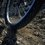# Need help with Mass-Energy EquivalenceIf mass-energy equivalence is taken into account when water is cooled to form ice, then:

Argument 1. The mass of the water should remain unchanged as the energy lost during the phase change is accounted by lowering of potential energy of the molecules of water.

Argument 2. When water is cooled to form ice, its thermal energy decreases. By mass-energy equivalence, mass should decrease.

Which one do you think is correct?Note by Lokesh Sharma
6 years, 8 months ago

This discussion board is a place to discuss our Daily Challenges and the math and science related to those challenges. Explanations are more than just a solution — they should explain the steps and thinking strategies that you used to obtain the solution. Comments should further the discussion of math and science.

When posting on Brilliant:

• Use the emojis to react to an explanation, whether you're congratulating a job well done , or just really confused .
• Ask specific questions about the challenge or the steps in somebody's explanation. Well-posed questions can add a lot to the discussion, but posting "I don't understand!" doesn't help anyone.
• Try to contribute something new to the discussion, whether it is an extension, generalization or other idea related to the challenge.

MarkdownAppears as
*italics* or _italics_ italics
**bold** or __bold__ bold
- bulleted- list
• bulleted
• list
1. numbered2. list
1. numbered
2. list
Note: you must add a full line of space before and after lists for them to show up correctly
paragraph 1paragraph 2

paragraph 1

paragraph 2

[example link](https://brilliant.org)example link
> This is a quote
This is a quote
    # I indented these lines
# 4 spaces, and now they show
# up as a code block.

print "hello world"
# I indented these lines
# 4 spaces, and now they show
# up as a code block.

print "hello world"
MathAppears as
Remember to wrap math in $$ ... $$ or $ ... $ to ensure proper formatting.
2 \times 3 $2 \times 3$
2^{34} $2^{34}$
a_{i-1} $a_{i-1}$
\frac{2}{3} $\frac{2}{3}$
\sqrt{2} $\sqrt{2}$
\sum_{i=1}^3 $\sum_{i=1}^3$
\sin \theta $\sin \theta$
\boxed{123} $\boxed{123}$

Sort by:

1

- 6 years, 8 months ago

i think 1st one is correct... if i am wrong then please tell me why other one is right

- 6 years, 8 months ago

This question was asked in AIEEE 2002. The answer key to this question to any place I have searched so far is supporting Argument 2.(The answer to this question is (c))

Take a look at this excerpt from Wikipedia regarding mass-energy equivalence: "E = mc2 has frequently been used as an explanation for the origin of energy in nuclear processes, but such processes can be understood as simply converting nuclear potential energy, without the need to invoke mass–energy equivalence."

So, the other way round it implies you can substitute mass-energy equivalence to explain energy released. This supports argument 2.

- 6 years, 8 months ago

plz explain...

- 6 years, 8 months ago

Explain what?

- 6 years, 8 months ago

Argument 2 is correct.

- 6 years, 8 months ago

1

- 6 years, 8 months ago